# IERT Entrance Exam Syllabus 2023: Polytechnic Exam Pattern in Hindi PDF Download

IERT Entrance Exam Syllabus 2023: The Institute of Engineering and Rural Technology [इंजीनियरिंग और ग्रामीण प्रौद्योगिकी संस्थान (IERT)] conducts entrance exams for admission into engineering, technology, and also other courses. The selection process for IERT admission is a written exam and an interview. The IERT Syllabus 2023 consists of topics in mathematics, physics, and chemistry. Students who qualify for the IERT Entrance Exam will be called for the next round, which is the interview. Check the Eligibility Criteria, Exam Pattern, and IERT Entrance Exam Syllabus PDF from the below section.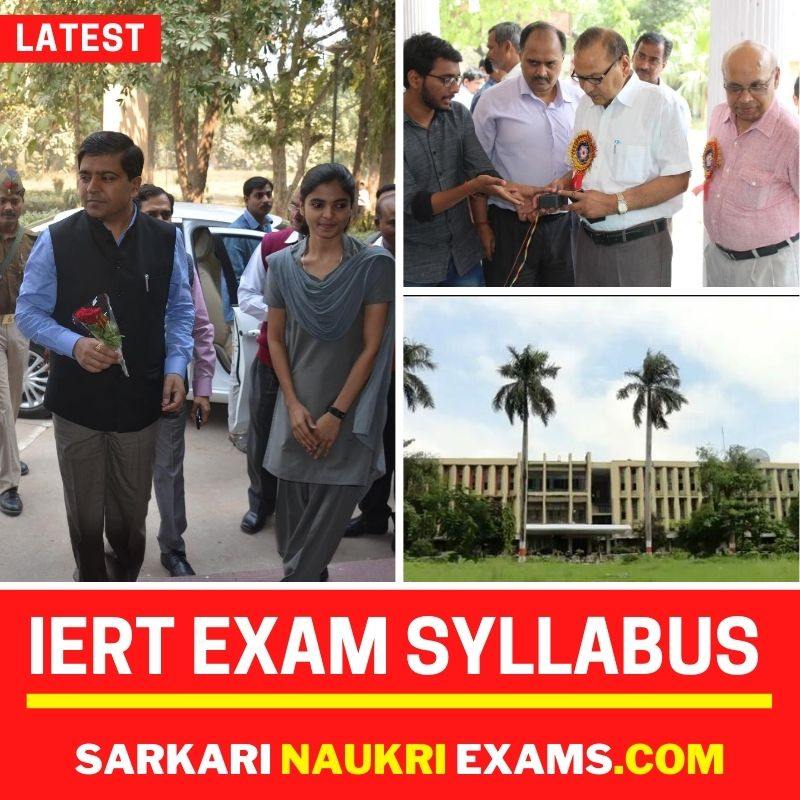✦ IERT प्रवेश परीक्षा 2023 के लिए पंजीकरण कुछ ही दिनों में शुरू होगा। नीचे से नवीनतम IERT प्रवेश परीक्षा सिलेबस 2023 की जाँच करें।

✦ Official Source

• iertonline.in
Contents-

## IERT Entrance Exam Syllabus 2023

IERT Entrance Exam Syllabus 2023 in Hindi PDF Download and Exam Pattern From the below section and article, we have provided a complete IERT Diploma Entrance Exam Syllabus 2023. Therefore, candidates who are willing to qualify for the IERT Entrance Examination 2023 should check all sections in this article. And you can download the IERT entrance exam syllabus 2023 pdf from a direct link at the end of the page.

## IERT Entrance Exam Syllabus/Pattern 2023

The IERT Entrance Exam 2023 will be conducted as 3 separate tests as per their applied courses, which are the Engineering Diploma (6 semesters), Management Diploma (4 semesters), and Post Diploma (3 semesters) in Computer Application Courses. The Institute of Engineering and Rural Technology officials are going to conduct the IERT Entrance Exam for the session 2023–24. The selection process for the IERT Exam is a written exam and an interview.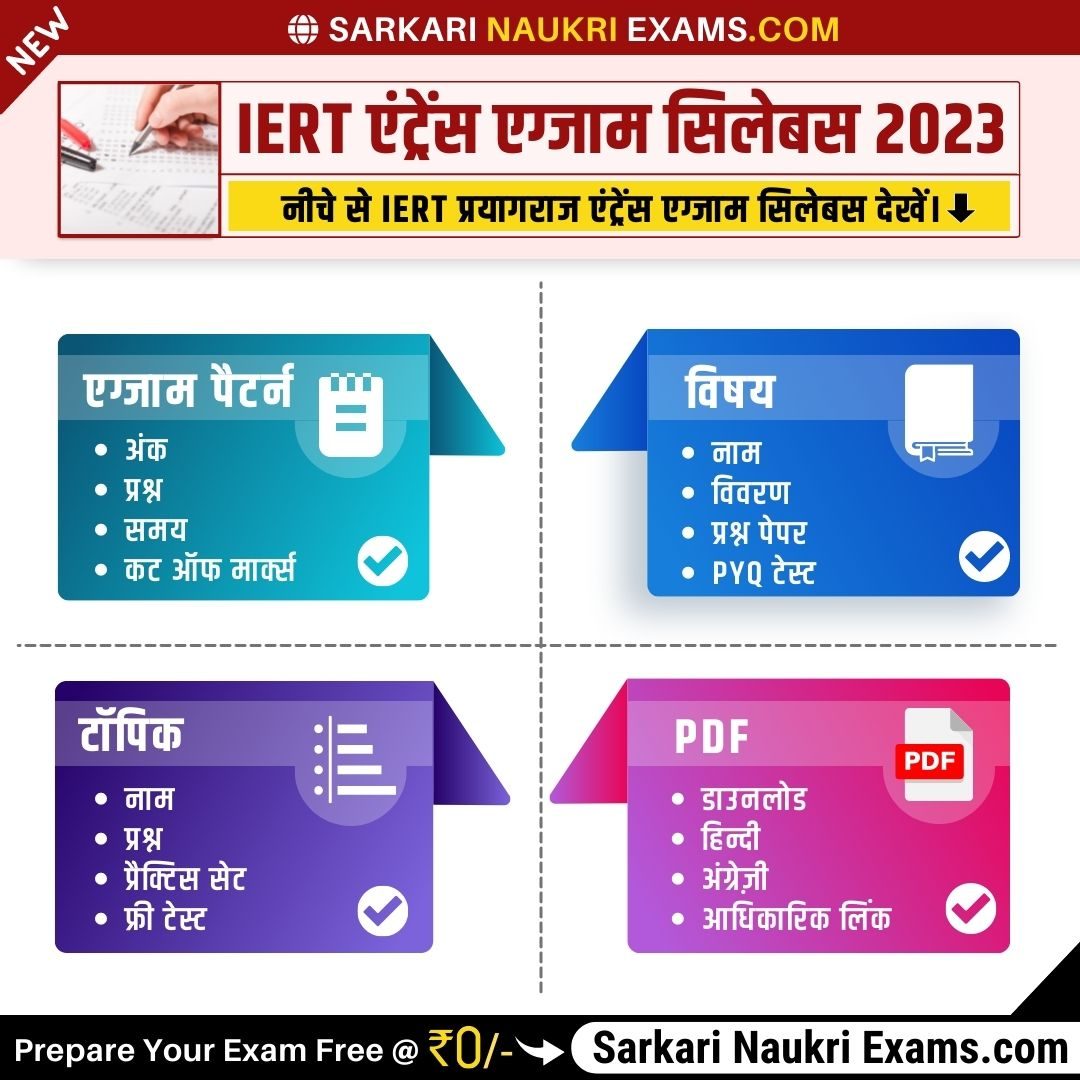Exam Pattern (परीक्षा पैटर्न)

## IERT Polytechnic Entrance Exam Syllabus Mathematics Topics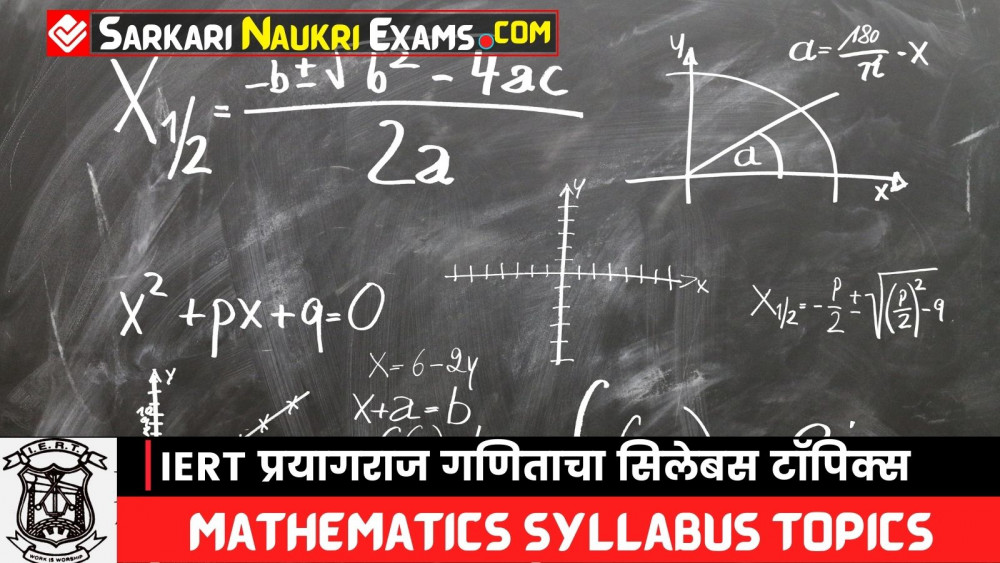Arithmetics (अंकगणित)

• Simple & Compound interest.
• Work-time and speed-time.
• Bank deposits and payments in installments, Taxation.
• Percentage, Profit, Loss & Discount.
• Ratio, Proportion & Mixture.

Statistics (सांख्यिकी)

• Importance of statistics and its usefulness.
• Classification of data frequency distribution, cumulative frequency. Graphical representation of statistical data, bar chart, histogram, frequency
polygon, frequency curves, cumulative frequency curve, and a graphical representation, Pie diagram.
• Measures of Central Tendency- Mean, Median & Mode.
• Measures of Dispersion – Range, Mean Deviation, Standard Deviation & Variance.

Algebra (बीजगणित)

• Number System-Natural Numbers, Whole Numbers, Integers, Even Numbers, Odd Numbers, Rational Numbers, Irrational Numbers, Real Numbers, Prime Numbers, Twin Prime Numbers, Composite Numbers, and their characteristics.
• Laws of Indices, Surds & Simplification.
• Square Roots & Cube Roots.
• Logarithms-definition, laws, and their application, to find the characteristics & mantissa.
• Factors-expressions in the form of the difference of two squares, trinomial expression, factorization by completing the square, expressions
in the form of sum and difference of two cubes, remainder theorem, and expression of the type a3+b3+c3-3abc.
• L.C.M. & H.C.M by factorization.
• The solution of simultaneous equations (linear equations with three variables) and problems based on them.
• Set Theory – Sets, Representation of Sets, Types of Sets, Subsets, Universal set, Complement of Set, Union of Sets, Interaction of Sets, Disjoint
Set, Difference of Sets, Laws of Algebra of Sets & Simple Problems with the help of Venn diagram.
• Mappings - Into Mapping, Onto Mapping, One-One Mapping, and Many-One Mapping.

Trigonometry (त्रिकोणमिति)

• Circular measures (relation between radian & degree), the definition of trigonometric ratios, and their relations.
• Trigonometrical ratios of angles of 00, 300, 400, 600, 900 and1800
• Trigonometrical ratios of angles of 90±θθ, 180±θθ, 270±θθ, 360±θθ, where θθ is any angle.
• Trigonometrical ratios (sine, cosine, tangent, and cotangent) for the sum & difference of two angles.
• Expressing products of sines and cosines into sum and difference form.
• Trigonometrical ratios of multiple & sub-multiple angles.
• The angle of elevation and depression. Simple problems with height & distance.

Geometry (ज्यामिति)

Pythagoras Theorem and its extension: Problems based on-

• The square on one side of a triangle is greater, equal, or smaller than the sum of the squares on two sides of it, according to as the angle between these two sides is an obtuse angle, a right angle, or an acute angle. In the case of an un-equality, the difference is twice the rectangle contained by one of these sides and the projection of the other on this site. Knowledge of the Pythagoras numbers (3, 4, 5), (5, 12, 13), etc.
• Apollonius Theorem- The sum of the squares contained on the two sides of a triangle equals twice the sum of the squares contained on half of
the third side and the median bisecting this site.

## IERT Polytechnic Exam Syllabus Physics TopicsMeasurement (माप)

• Fundamental units: Fundamental quantities, S.I. system of fundamental units, standard meter, standard kilograms, standard second, micron, Angstrom & light-years derived units.
• Measurements of small length: Principal of vernier calipers & screw gauge and its applications, limit of accuracy of measurement, significant figures.

Kinematics (गतिकी)

Elementary Knowledge of Vectors-

• Distance & displacement, speed & velocity, representation of a vector, the addition of vectors (triangle & polygon method-only force diagram).

Acceleration

• Equation of motion under gravity (straight line).

Mechanics (मैकेनिक्स)

Laws of Motion-

• Newton’s law of motion, the first law, the second law of motion (force mass acceleration), the unit of force (Newton & Kilogram weight), the law of conservation of momentum, the third law of motion.

Moment of Force-

• Moment of force and its unit, the principle of moments, the equilibrium of a body under the system of parallel forces, the center of gravity, condition of stable, unstable, and neutral equilibrium.

## IERT Entrance Exam Syllabus Chemistry Topics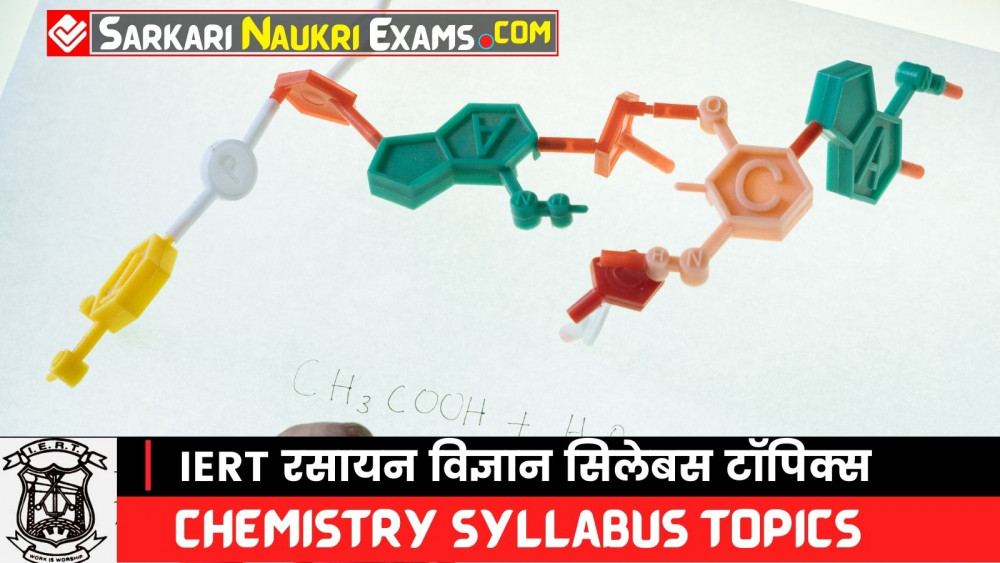General and Physical Chemistry (सामान्य और भौतिक रसायन विज्ञान)

• Science & scientific method, the definition of chemistry, branches of chemistry, and the importance of chemistry in the modern age.
• Matter and its three physical states & deviation based on molecular kinetic theory.
• Atom, molecule, and laws of chemical combination (Statement and examples).
• Atomic structure, nuclear proton, neutron, atomic mass unit, electrons and their orbits (Atomic no. & isotopes) Introduction of radioactivity and nuclear energy.
• Valency- electrovalent and co-valency.
• Symbol, formula, chemical equation, and balancing of simple chemical equations.
• Gas laws – Boyle's laws, Charles's law, and gas equation; Gay-lussac’s law of gaseous volumes, Dalton’s law of partial pressure, and Grahm’s law of diffusion.
• Avogadro’s Hypothesis and its importance.
• Atomic weight, molecular weight & equivalent weight. Examples and methods of their determinations (for molecular weight only Victor Meyer’s and Hoffman's
method).
• Faradays the law of electrolysis, explanation of acid, base & salt, and the elementary concept of ionization. Principles of a simple voltaic cell.
• Different chemical reactions-addition, substitution, dissociation, hydrolysis, double decomposition, oxidation-reduction, and catalytic.
• Explanation of solution-solution, solute, solvent saturated solution, unsaturated solution, supersaturated solution, normal solution-molal solution, molar solution,
solubility, viz; the reaction of the solution, some important solvents water, benzene, ether, acetone, carbon tetrachloride, and Alcohol.

IERT Syllabus: Inorganic Chemistry [अकार्बनिक रसायन शास्त्र]

• Classification of elements, general characteristics of Mendeleev's periodic table.
• Electronic configuration & position of the following elements in the periodic table, hydrogen, carbon, nitrogen, phosphorus, sulfur &chlorine.
• Drinking water, the volumetric composition of water, hard & soft water, temporary & permanent hard water. Physical & chemical methods for the removal of hardness of
the water.
• Laboratory method of preparation; properties and uses of the following gases; oxygen, nitrogen, chlorine, ammonia, sulfur dioxide, sulphurated hydrogen&hydrogen
chloride.
• Difference between metals & nonmetals, the metallurgy of Aluminum.

IERT Syllabus: Organic Chemistry [और्गॆनिक रसायन]

• Introduction of organic chemistry. Definition and scope of organic chemistry, the difference between organic & inorganic compounds. Preliminary introduction of
tetrahedral nature of carbon atoms.
• Classification & nomenclature of organic compounds-Aliphatic & Aromatic (Homologous Series).
• Methods of preparation and properties of Paraffins (Methane and Ethane) and unsaturated hydrocarbons (Ethylene and acetylene).
• Simple introduction of Plastic, artificial fiber, medicines, explosives, soap, and detergents.

IERT Syllabus: Numerical Problem [संख्यात्मक समस्या]

• Numerical problems based on the following topics – laws of chemical combination, percentage composition, empirical and molecular formula, the chemical equation,
• equivalent weight, valency, the molecular weight of Volatile substances, Graham’s Law of diffusion, and Gas equation.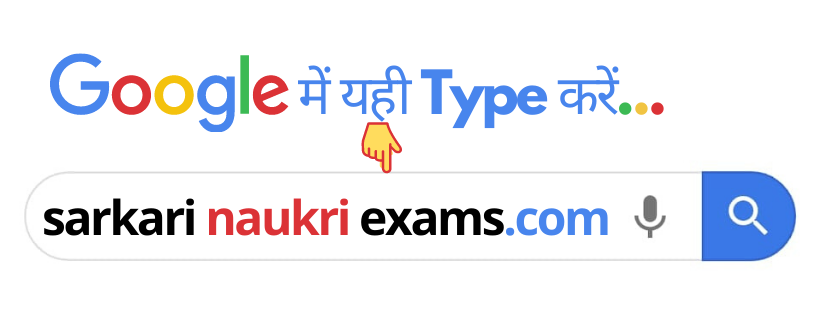### IERT Entrance Exam Syllabus 2023 FAQs

⭐What is the marking scheme of the IERT Entrance Exam?

⭐How many questions will be asked in the IERT Entrance Exam?

There will be 75 questions in the IERT Entrance Exam

⭐Can I get free practice mock test for this exam syllabus?

Yes, you can get the free mock test from the Sarkari Naukri Exams.com

⭐Is there negative marking for the exam syllabus?

Yes, there is negative marking for this exam.

#### Comment

×

A

Anuj Patel- IERT ka pcm ka notes chahiye sir please

Sarkari Naukri Exams- Hi Anuj patel,

हम स्टडी नोट्स और पिछले पेपर पर काम कर रहे हैं। आपको पहली अध्ययन सामग्री Previous Year Paper अगले सप्ताह तक मिल जाएगी।

Regards,
Sarkari Naukri Exams Team!

Y

Yuvraj Patel- Pura notes chahiye

Sarkari Naukri Exams- Hi Yuvraj Patel,

Thanks for the feedback!!

Regards,
Sarkari Naukri Exams Team!

P

Pankaj Kumar Maurya- Iert exam Hindi or English me hora hai

Sarkari Naukri Exams- Hi Pankaj Kumar maurya,

You will get both options (Hindi & English) at your ease. (आपको आसानी से दोनों विकल्प (हिंदी और अंग्रेजी) मिलेंगे।)

Regards,
Sarkari Naukri Exams Team!

K

Sarkari Naukri Exams- Hi Karan Sahu,

Regards,
Sarkari Naukri Exams Team!

Updated: